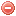# Definition

The daylight hours are maximum possible duration of sunshine for a given day of the year (Allen et al. 1998).

# Formula

## Formulation according to FAO:

The daylight hours $$N$$ are obtained as follows (Allen et al. 1998):

$N=\dfrac{24}{\pi}\cdot\omega_s$

where $$\omega_s$$ is the sunset hour angle [rad], which is calculated as follows:

$\omega_s=\arccos [-\tan\varphi\cdot\tan\delta]$

where $$\delta$$ is the solar declination [rad], and $$\varphi$$ the latitude [rad].

The solar declination $$\delta$$ is calculated as follows:

$\delta=0.409\cdot\sin\bigg(\dfrac{2\pi}{365}\cdot J-1.39\bigg)$

where $$J$$ is the number of the day in the year between 1 (1 January) and 365 or 366 (31 December).

The conversion from decimal degrees to radians is obtained as follows:

$\mbox{[Radians]}=\dfrac{\pi}{180} \mbox{ [decimal degrees]}$

NB: for calculating potential evapotranspiration according to Thornthwaite, a daylight coefficient $$C$$ is required, which corresponds to the duration of sunlight in units of 12 hours. This daylight coefficient $$C$$ is thus given by:

$C=\dfrac{N}{12}$

## Formulation according to NFDRS

The procedure for determining daylight hours $$N_{nfdrs}$$ proposed by (Cohen & Deeming (1985) in order to calculate some components of the NFDRS is slightly different from that proposed by (Allen et al. (1998):

$N_{nfdrs}=24\cdot\bigg(1-\dfrac{\arccos(\tan\varphi\cdot\tan\delta)}{\pi}\bigg)$

where $$\varphi$$ is latitude [rad] and $$\delta$$ the solar declination [rad].

The latitude $$\varphi$$ [rad] is given by:

$\varphi=\varphi_{deg}\cdot{0.01745}$

where $$\varphi_{deg}$$ is the latitude in decimal degrees.

The solar declination $$\delta$$ is calculated as follows:

$\delta=0.41008\cdot\sin\Big((J-82)\cdot{0.01745}\Big)$

where $$J$$ is the Julian date.

# Reference

Cohen & Deeming (1985)
Allen et al. (1998)

### Bibliography SearchNo results!

No results were found. Are you sure you searched for a tag?

### Symbols

 Variable Description Unit $$T$$ air temperature °C $$T_{dew}$$ dew point temperature °C $$H$$ air humidity % $$P$$ rainfall mm $$U$$ windspeed m/s $$w$$ days since last rain (or rain above threshold) d $$rr$$ days with consecutive rain d $$\Delta t$$ time increment d $$\Delta{e}$$ vapor pressure deficit kPa $$e_s$$ saturation vapor pressure kPa $$e_a$$ actual vapor pressure kPa $$p_{atm}$$ atmospheric pressure kPa $$PET$$ potential evapotranspiration mm/d $$r$$ soil water reserve mm $$r_s$$ surface water reserve mm $$EMC$$ equilibrium moisture content % $$DF$$ drought factor - $$N$$ daylight hours hr $$D$$ weighted 24-hr average moisture condition hr $$\omega$$ sunset hour angle rad $$\delta$$ solar declination rad $$\varphi$$ latitude rad $$Cc$$ cloud cover Okta $$J$$ day of the year (1..365/366) - $$I$$ heat index - $$R_n$$ net radiation MJ⋅m-2⋅d-1 $$R_a$$ daily extraterrestrial radiation MJ⋅m-2⋅d-1 $$R_s$$ solar radiation MJ⋅m-2⋅d-1 $$R_{so}$$ clear-sky solar radiation MJ⋅m-2⋅d-1 $$R_{ns}$$ net shortwave radiation MJ⋅m-2⋅d-1 $$R_{nl}$$ net longwave radiation MJ⋅m-2⋅d-1 $$\lambda$$ latent heat of vaporization MJ/kg $$z$$ elevation m a.s.l. $$d_r$$ inverse relative distance Earth-Sun - $$\alpha$$ albedo or canopy reflection coefficient - $$\Delta$$ slope of the saturation vapor pressure curve kPa/°C $$Cc$$ cloud cover eights $$ROS$$ rate of spread m/h $$RSF$$ rate of spread factor - $$WF$$ wind factor - $$WRF$$ water reserve factor - $$FH$$ false relative humidity - $$FAF$$ fuel availability factor - $$PC$$ phenological coefficient -

 Suffix Description $$-$$ mean / daily value $$_{max}$$ maximum value $$_{min}$$ minimum value $$_{12}$$ value at 12:00 $$_{13}$$ value at 13:00 $$_{15}$$ value at 15:00 $$_{m}$$ montly value $$_{y}$$ yearly value $$_{f/a}$$ value at fuel-atmosphere interface $$_{dur}$$ duration $$_{soil}$$ value at soil level

 Constant Description $$e$$ Euler's number $$\gamma$$ psychrometric constant $$G_{SC}$$ solar constant $$\sigma$$ Stefan-Bolzmann constant